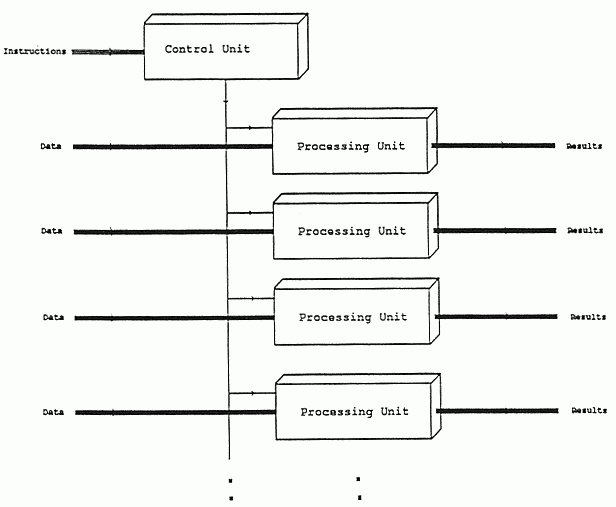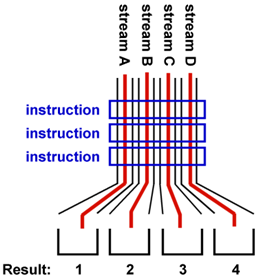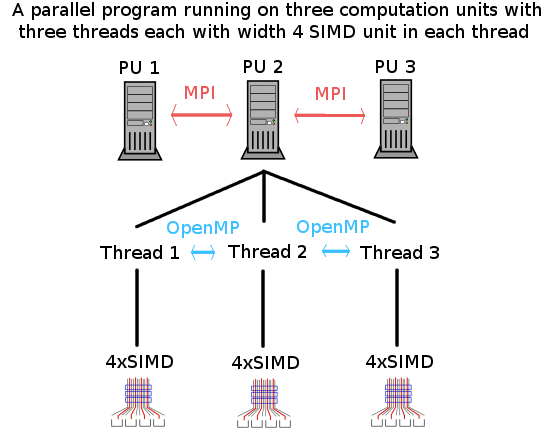## 2.0 What is SIMD?

SIMD stands for Symmetric Instructions and Multiple Data. The same set of instructions is executed in parallel to different sets of data. This reduces the amount of hardware control logic needed by N times for the same amount of calculations, where N is the width of the SIMD unit. SIMD computation model is illustrated in figure 1.

 Figure 1The huge downside of SIMD is that the N paths can not be processed differently while in real life algorithms there will be need to process different data differently. This kind of path divergence is handled in SSE either by multiple passes with different masks or by reverting to processing each path in scalar. If large proportion of an algorithm can be run without divergence then SSE can give benefit. Generally, the larger the SIMD width N, the bigger the pain of getting all the speed out of the processing units.

Another illustration of SIMD (copied from Intel) is pictured in figure 2. It underlines the vertical nature of most SSE operations. This concept of data being horizontally for SSE will be utilized through this guide.

 Figure 2#### SIMD compared to other levels of parallel computing

In shared memory (thread) level parallelization different parallel paths can execute completely unique set of instructions. This makes for a much simpler parallel programming for example through an API like OpenMP. This is also true for parallelization between different calculation units without shared memory. However, then usually communication has to be coded in manually through an interface like MPI.

Both of those are MIMD or multiple data and multiple instructions. Note that all of these parallel concepts can and should be utilized at the same time. For example MPI can be used to divide a job between computation units. Then OpenMP used to divide a part of the job between available threads. Finally vectorization can be utilized inside each thread. This is illustrated in figure 3:

 Figure 3